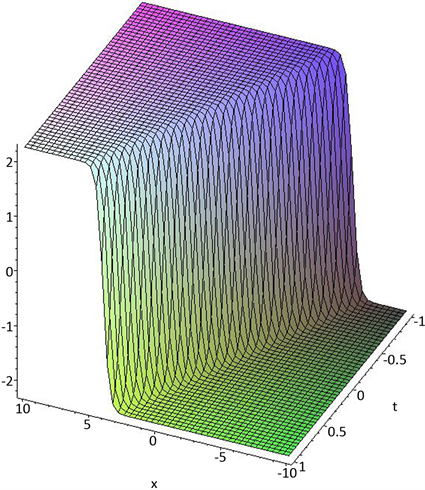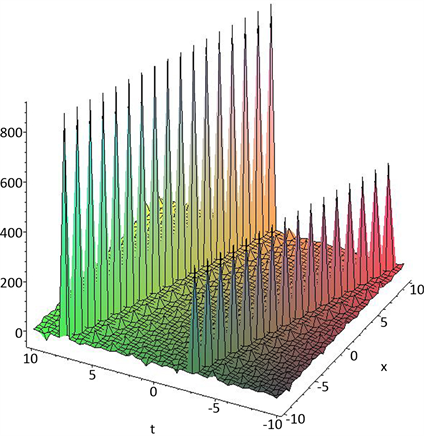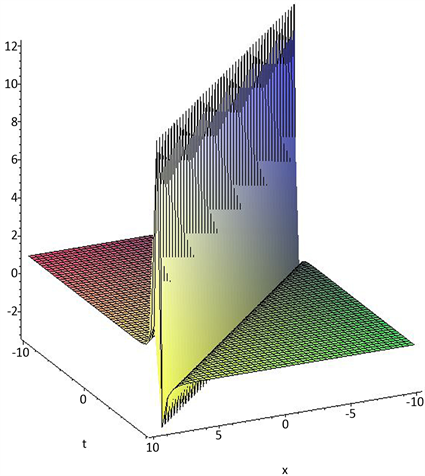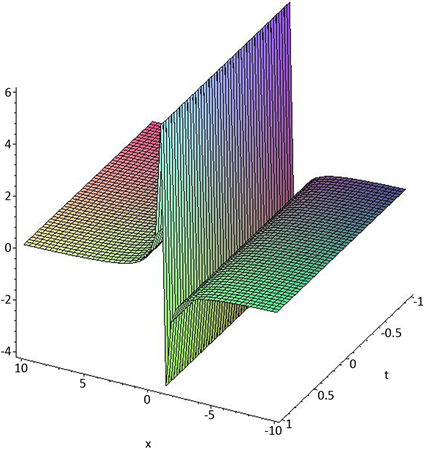# 利用exp(-G(ξ))方法和拟设函数法求Sharma-Tasso-Olver方程精确解The Exact Solutions of the Sharma-Tasso-Olver Equation Using exp(-G(ξ)) Method and Ansatz Method

DOI: 10.12677/AAM.2019.82025, PDF, HTML, XML, 下载: 415  浏览: 1,765

Abstract: Using the traveling wave transformation and homogeneous balance simplified the Shar-ma-Tasso-Olver equation to obtain the reduced ordinary differential equations, using the exp(-G(ξ))-method to get the trigonometric function solution, hyperbolic function solution and the rational function solution. In addition, an exact soliton solution is provided by using the Ansatz method.

1. 引言

${u}_{t}+3\epsilon {u}^{2}{u}_{x}+3\epsilon {u}_{x}^{2}+3\epsilon u{u}_{xx}+\epsilon {u}_{xxx}=0$ (1)

2. $exp\left(-G\left(\xi \right)\right)$ 方法

$u\left(x,t\right)=u\left(\xi \right)$ , $\xi =kx-wt$ ,

$-w{u}^{\prime }+3\epsilon k{u}^{2}{u}^{\prime }+3\epsilon {k}^{2}{\left({u}^{\prime }\right)}^{2}+3\epsilon {k}^{2}u{u}^{″}+\epsilon {k}^{3}{u}^{‴}=0,$

$-wu+\epsilon k{u}^{3}+3\epsilon {k}^{2}u{u}^{\prime }+\epsilon {k}^{3}{u}^{″}=0.$ (2)

$u\left(\xi \right)=\underset{n=0}{\overset{m}{\sum }}{a}_{n}{\left(\mathrm{exp}\left(-G\left(\xi \right)\right)\right)}^{n},$ (3)

${G}^{\prime }\left(\xi \right)=\mathrm{exp}\left(-G\left(\xi \right)\right)+\mu \mathrm{exp}\left(G\left(\xi \right)\right)+\lambda ,$ (4)

$u\left(\xi \right)={a}_{0}+{a}_{1}\mathrm{exp}\left(-G\left(\xi \right)\right).$ (5)

$\mathrm{exp}{\left(-G\left(\xi \right)\right)}^{3}:2{k}^{3}\epsilon {a}_{1}-3\epsilon {k}^{2}{a}_{1}^{2}+k\epsilon {a}_{1}^{3}=0,$ (6)

$\mathrm{exp}{\left(-G\left(\xi \right)\right)}^{2}:{k}^{3}\epsilon {a}_{1}-{k}^{2}\epsilon \lambda {a}_{1}^{2}+{k}^{2}\epsilon {a}_{1}^{2}{a}_{0}=0,$ (7)

$\mathrm{exp}\left(-G\left(\xi \right)\right):{k}^{3}\epsilon {\lambda }^{2}{a}_{1}+2{k}^{3}\epsilon \mu {a}_{1}-3{k}^{2}\epsilon \mu {a}_{1}^{2}-3{k}^{2}\epsilon \lambda {a}_{1}{a}_{0}+3k\epsilon {a}_{1}{a}_{0}^{2}-w{a}_{1}=0,$ (8)

$\mathrm{exp}{\left(-G\left(\xi \right)\right)}^{0}:{k}^{3}\epsilon \lambda \mu {a}_{1}+3{k}^{2}\epsilon \mu {a}_{0}+k\epsilon {a}_{0}^{3}-w{a}_{0}=0.$ (9)

① 当 ${\lambda }^{2}-4\mu >0$ ，且 $\mu \ne 0$ 时，

${u}_{1.1}=\frac{2k\mu }{-\sqrt{{\lambda }^{2}-4\mu }\mathrm{tanh}\left(\sqrt{{\lambda }^{2}-4\mu }/2\left(\xi +C\right)\right)-\lambda }+\frac{\lambda k}{2}$(如图1)

② 当 ${\lambda }^{2}-4\mu <0$ ，且 $\mu \ne 0$ 时，

${u}_{1.2}=\frac{2k\mu }{\sqrt{4\mu -{\lambda }^{2}}\mathrm{tan}\left(\sqrt{4\mu -{\lambda }^{2}}/2\left(\xi +C\right)\right)-\lambda }+\frac{\lambda k}{2}$(如图2)

③ 当 ${\lambda }^{2}-4\mu >0$ ，且 $\mu =0,\lambda \ne 0$ 时，

${u}_{1.3}=\frac{\lambda k}{\mathrm{cosh}\left(\lambda \left(\xi +C\right)\right)+\mathrm{sinh}\left(\lambda \left(\xi +C\right)\right)-1}+\frac{\lambda k}{2}$(如图3)

④ 当 ${\lambda }^{2}-4\mu =0$ ，且 $\mu \ne 0,\lambda \ne 0$ 时，

${u}_{1.4}=-\frac{{\lambda }^{2}k\left(\xi +C\right)}{2\lambda \left(\xi +C\right)+4}+\frac{\lambda k}{2}$(如图4)

⑤ 当 ${\lambda }^{2}-4\mu =0$ ，且 $\mu =0,\lambda =0$ 时，

${u}_{1.5}=\frac{k}{\xi +C}+\frac{\lambda k}{2}$(如图5)Figure 1. Hyperbolic function solution ${u}_{1.1}$Figure 2. Trigonometric function solution ${u}_{1.2}$Figure 3. Hyperbolic function solution ${u}_{1.3}$Figure 4. Rational function solution ${u}_{1.4}$Figure 5. Rational function solution ${u}_{1.5}$

① 当 ${\lambda }^{2}-4\mu >0$ ，且 $\mu \ne 0$ 时，

${u}_{2.1}=\frac{4k\mu }{-\sqrt{{\lambda }^{2}-4\mu }\mathrm{tanh}\left(\sqrt{{\lambda }^{2}-4\mu }/2\left(\xi +C\right)\right)-\lambda }+\lambda k$

② 当 ${\lambda }^{2}-4\mu <0$ ，且 $\mu \ne 0$ 时，

${u}_{2.2}=\frac{4k\mu }{\sqrt{4\mu -{\lambda }^{2}}\mathrm{tan}\left(\sqrt{4\mu -{\lambda }^{2}}/2\left(\xi +C\right)\right)-\lambda }+\lambda k$

③ 当 ${\lambda }^{2}-4\mu >0$ ，且 $\mu =0,\lambda \ne 0$ 时，

${u}_{2.3}=\frac{2\lambda k}{\mathrm{cosh}\left(\lambda \left(\xi +C\right)\right)+\mathrm{sinh}\left(\lambda \left(\xi +C\right)\right)-1}+\lambda k$

④ 当 ${\lambda }^{2}-4\mu =0$ ，且 $\mu \ne 0,\lambda \ne 0$ 时，

${u}_{2.4}=-\frac{{\lambda }^{2}k\left(\xi +C\right)}{\lambda \left(\xi +C\right)+2}+\lambda k$

⑤ 当 ${\lambda }^{2}-4\mu =0$ ，且 $\mu =0,\lambda =0$ 时，

${u}_{2.5}=\frac{2k}{\xi +C}+\lambda k$

3. 拟设双曲函数法

$u\left(x,t\right)=A\mathrm{tan}{h}^{″}\tau$$\tau =B\left(x-vt\right)$(10)

${u}_{t}=-nvAB\left({\mathrm{tanh}}^{n-1}\tau -{\mathrm{tanh}}^{n+1}\tau \right),$ (11)

${u}_{x}=nvAB\left({\mathrm{tanh}}^{n-1}\tau -{\mathrm{tanh}}^{n+1}\tau \right),,$ (12)

${u}_{xx}=n\left(n-1\right)A{B}^{2}{\mathrm{tanh}}^{n-2}\tau -2{n}^{2}A{B}^{2}{\mathrm{tanh}}^{n}\tau +n\left(n+1\right)A{B}^{2}{\mathrm{tanh}}^{n+2}\tau ,$ (13)

$\begin{array}{c}{u}_{xxx}=n\left(n-1\right)\left(n-2\right)A{B}^{3}{\mathrm{tanh}}^{n-3}\tau -\left[n\left(n-1\right)\left(n-2\right)+2{n}^{3}\right]A{B}^{3}{\mathrm{tanh}}^{n-1}\tau \\ \text{\hspace{0.17em}}\text{\hspace{0.17em}}+\left[n\left(n+1\right)\left(n+2\right)+2{n}^{3}\right]A{B}^{3}{\mathrm{tanh}}^{n+1}\tau -n\left(n+1\right)\left(n+2\right)A{B}^{3}{\mathrm{tanh}}^{n+3}\tau ,\end{array}$ (14)

$\begin{array}{l}\text{ }-nvAB\left({\mathrm{tanh}}^{n-1}\tau -{\mathrm{tanh}}^{n+1}\tau \right)+3\epsilon nv{A}^{2}B\left({\mathrm{tanh}}^{2n-1}\tau -{\mathrm{tanh}}^{2n+1}\tau \right)\\ \text{ }+3\epsilon {\left[nvAB\left({\mathrm{tanh}}^{n-1}\tau -{\mathrm{tanh}}^{n+1}\tau \right)\right]}^{2}+3\epsilon {A}^{2}{B}^{2}\left[n\left(n-1\right){\mathrm{tanh}}^{2n-2}\tau \\ \text{ }-2{n}^{2}{\mathrm{tanh}}^{2n}\tau n\left(n+1\right){\mathrm{tanh}}^{2n+2}\tau \right]+\epsilon \left[n\left(n-1\right)\left(n-2\right)A{B}^{3}{\mathrm{tanh}}^{n-3}\tau \\ \text{ }-\left(n\left(n-1\right)\left(n-2\right)+2{n}^{3}\right)A{B}^{3}{\mathrm{tanh}}^{n-1}\tau +\left(n\left(n+1\right)\left(n+2\right)+2{n}^{3}\right)A{B}^{3}{\mathrm{tanh}}^{n+1}\tau \\ \text{ }-n\left(n+1\right)\left(n+2\right)A{B}^{3}{\mathrm{tanh}}^{n+3}\tau .\end{array}$ (15)

$B=A$ 或者 $B=\frac{A}{2}$$v=\epsilon {A}^{2}$

$u\left(x,t\right)=A\mathrm{tanh}\left(B\left(x-vt\right)\right)$

4. 结论

  Shang, Y.D., Huang, Y. and Yuan, W.J. (2011) Bäcklund Transformations and Abundant Exact Explicit Solutions of the Sharma-Tasso-Olver Equation. Applied Mathematics and Computation, 217, 7172-7183. https://doi.org/10.1016/j.amc.2011.01.115  Fan, E.G. and Zhang, H.Q. (1998) A New Approach to Bäcklund Transformations of Nonlinear Evolution Equations. Applied Mathematics and Mechanics, 19, 645-650.  Shang, Y. (2008) The Extended Hyperbolic Functions Method and Exact Solutions to the Long-Short Wave Resonance Equations. Chaos, Solitons & Fractals, 36, 762-771. https://doi.org/10.1016/j.chaos.2006.07.007  Wang, S., Tang, X.Y. and Lou, S.Y. (2004) Soliton Fission and Fusion: Burgers Equation and Sharma-Tasso-Olver Equation. Chaos, Solitons & Fractals, 21, 231-239. https://doi.org/10.1016/j.chaos.2003.10.014  Yan, Z.Y. (2003) Integrability of Two Types of the (2 + 1)-Dimensional Generalized Sharma-Tasso-Olver Integro-Differential Equations. Acta Mathematica Scientia, 22, 302-324.  Shang, Y.D., Qin, J.H. and Huang, Y. (2008) Abundant Exact and Explicit Solitary Wave and Periodic Wave Solutions to the Sharma-Tasso-Olver Equation. Applied Mathematics and Computation, 202, 532-538. https://doi.org/10.1016/j.amc.2008.02.034  Kudryashov, N.A. and Loguinova, N.B. (2008) Extended Simplest Equation Method for Nonlinear Differential Equations. Applied Mathematics and Computation, 205, 396-402.  Bekir, A. and Boz, A. (2008) Exact Solutions for Nonlinear Evolution Equations Using Exp-Function Method. Physics Letters A, 372, 1619-1625. https://doi.org/10.1016/j.physleta.2007.10.018  Erbas, B. and Yusufoglu, E. (2009) Exp-Function Method for Constructing Exact Solutions of Sharma-Tasso-Olver Equation. Chaos, Solitons & Fractals, 41, 2326-2330. https://doi.org/10.1016/j.chaos.2008.09.003  Lian, Z.J. and Lou, S.Y. (2005) Symmetries and Exact Solutions of the Sharma-Tasso-Olver Equation. Nonlinear Anal, 63, e1167-e1177.  Inan, I.E. and Kaya, D. (2007) Exact Solutions of Some Nonlinear Partial Differential Equations. Physica A, 381, 104-115. https://doi.org/10.1016/j.physa.2007.04.011  Wazwaz, A.M. (2007) New Solitons and Kinks Solutions to the Sharma-Tasso-Olver Equation. Applied Mathematics and Computation, 188, 1205-1213. https://doi.org/10.1016/j.amc.2006.10.075  Akbulut, A., Kaplan, M. and Tascan, F. (2017) The Investigation of Exact Solutions of Nonlinear Partial Differential Equations by Using Method. Optik, 132, 382-387. https://doi.org/10.1016/j.ijleo.2016.12.050  Roshid, H.O., Alam, M.N. and Akbar, M.A. (2015) Exact Trav-eling Wave Solutions to the (3+1)-Dimensional mKdV –ZK and the (2+1)-Dimensional Burgers Equations via -Expansion Method. Alexandria Engineering Journal, 54, 635-644. https://doi.org/10.1016/j.aej.2015.05.005  Bhrawy, A.H. and Obaid, M. (2012) New Exact Solutions for the Zhiber-Shabat Equation Using the Extended F-Expansion Method. Life Sciences, 9, 1154-1162.  Fan, E.G. (2000) Two New Applications of the Homogeneous Balance Method. Physics Letters A, 265, 353-357. https://doi.org/10.1016/S0375-9601(00)00010-4  Zhang, H.Q. (2001) Extension and Application of Homogenous Balance Method. Applied Mathematics and Mechanics, 21, 321-325.  Jawad, A.J.M., Petkovic, M.D. and Biswas, A. (2010) Soliton Solutions of Burgers Equations and Perturbed Burgers Equation. Applied Mathematics and Computation, 216, 3370-3377. https://doi.org/10.1016/j.amc.2010.04.066  Wang, G.W. and Kara, A.H. (2018) Group Analysis, Fractional Explicit Solutions and Conservation Laws of Time Fractional Generalized Burgers Equation. Communications in Theoretical Physics, 69, 5-8.